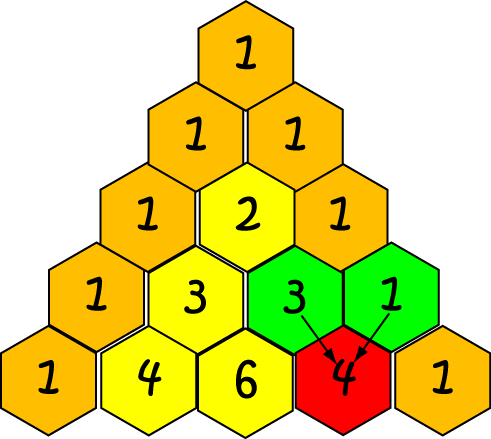# Definition of Pascal's TrianglePascal's Triangle is a number pattern in which the numbers for the rows of a triangle. The numbers along the two outside edges are all $1$s. Each of the inside numbers is obtained by adding the two numbers that are diagonally above it in the triangle together.

In the diagram, the numbers in green hexagons are diagonally above the number in the red hexagon. They are added together to give $3 + 1 = 4$, which is the number in the red hexagon.

Pascal's triangle has many interesting properties. For example, it has a vertical axis of symmetry.

The numbers in the $(n + 1)$st row of Pascal's triangle form the coefficients for the expansion of $(a + b)^n$.

### Description

The aim of this dictionary is to provide definitions to common mathematical terms. Students learn a new math skill every week at school, sometimes just before they start a new skill, if they want to look at what a specific term means, this is where this dictionary will become handy and a go-to guide for a student.

### Audience

Year 1 to Year 12 students

### Learning Objectives

Learn common math terms starting with letter P

Author: Subject Coach
You must be logged in as Student to ask a Question.